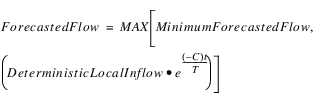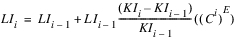Objects and Methods : Reach : User Methods : Generate Local Inflows
Generate Local Inflows
This category contains methods that forecast the Local Inflow based on known inflow values. You input the historical inflows to the reach, and the methods adjust the values to represent a forecast.
The methods in this category execute at the beginning of each timestep. This category is dependent on having either the Specify Local Inflow, Solve Inflow or Outflow; or the Specify Local Inflow, Solve Outflow method selected.None
This method is the default for this category but does nothing.Geometric Recession
On each timestep in the forecast period, this method will adjust the inflow hydrographs, input in the Deterministic Local Inflow slot, and write the results to the Local Inflow series slot.
Slots Specific to This Method
Deterministic Local Inflow
Type: Series
Units: FLOW
Description: This slots holds a timeseries of the actual Local Inflows to the reach. These values are then adjusted by the forecast method and set on the Local Inflow slot.
Information: At the end of the run, the Local Inflow slot will be identical to this slot.
I/O: Input only
Period of Perfect Knowledge
Type: Scalar
Units: FLOW
Description: Number of timesteps for which the forecast will equal the Deterministic Local Inflow; that is, the forecast is known with complete certainty.
Information: Minimum value of 1; maximum value equal to the number of timesteps in the forecast period.
I/O: Input only
Recession Factor
Type: Scalar
Units: NONE
Description: A decimal value that is multiplied by the previous Local Inflow to determine the current value after the Period of Perfect Knowledge.
Information:
I/O: Input only
Method Details
The Deterministic Local Inflow slot values are input for each timestep on a reach. At the beginning of each timestep, the Geometric Recession method is selected. For each forecast timestep within the period of perfect knowledge, the Local Inflow is set to the Deterministic Local Inflow value. For each forecast timestep after the period of perfect knowledge, the Local Inflow is set by multiplying the value of the Local Inflow from the previous timestep by the constant recession factor.
A value for the Deterministic Local Inflow slot must be known at every timestep during the run. If forecasted Local Inflow values are desired past the end of the run, there must also be values in the Deterministic Local Inflow slot at timesteps past the end of the run. If values for Deterministic Local Inflow are not entered past the end of the run, the Local Inflows for these timesteps are assumed to be zero.Exponential Recession
On each timestep in the forecast period, this method will adjust the inflow hydrographs, input in the Deterministic Local Inflow slot, and write the results to the Local Inflow series slot. The slot values of the Local Inflow will be the same as those in the Deterministic Local Inflow slot during the period of perfect knowledge. After the period of perfect knowledge, the Local Inflow slot values are recessed by a recession equation using the Deterministic Local Inflow slot value on the last day of perfect knowledge.
Slots Specific to This Method
Deterministic Local Inflow
Type: Series
Units: FLOW
Description: This slots holds a timeseries of the actual Local Inflows to the reach. These values are then adjusted by the forecast method and set on the Local Inflow slot.
Information: At the end of the run, the Local Inflow slot will be identical to this slot.
I/O: Input only
Forecast Period
Type: Table
Units: NONE
Description: Number of timesteps, not including the current timestep, that the inflow hydrograph will be adjusted.
Information:
I/O: Input only
Period of Perfect Knowledge
Type: Scalar
Units: FLOW
Description: Number of timesteps for which the forecast will equal the Deterministic Local Inflow; that is, the forecast is known with complete certainty.
Information: Minimum value of 1; maximum value equal to the number of timesteps in the forecast period.
I/O: Input only
Minimum Forecasted Flow
Type: Series
Units: FLOW
Description: The minimum forecasted flow.
Information: If the computed value for Local Inflow is less than the Minimum Forecasted Flow, it is set to the Minimum Forecasted Flow.
I/O: Input only
Low Flow Threshold
Type: Scalar
Units: FLOW
Description: The flow rate that dictates whether to use the Low Flow Recession Coefficient or the High Flow Recession Coefficient.
Information:
I/O: Input only
Low Flow Recession Coefficient
Type: Scalar
Units: NONE
Description: The recession coefficient used when the Deterministic Local Inflow (at the end of the Period of Perfect Knowledge) is below or equal to the Low Flow Threshold.
Information:
I/O: Input only
High Flow Recession Coefficient
Type: Scalar
Units: NONE
Description: The recession coefficient used when the Deterministic Local Inflow (at the end of the Period of Perfect Knowledge) is above the Low Flow Threshold.
Information:
I/O: Input only
Method Details
The Deterministic Local Inflow slot values are input for each timestep on a reach. At the beginning of each timestep, the Exponential Recession method is selected. For each forecast timestep within the period of perfect knowledge, the Local Inflow is set to the Deterministic Local Inflow value. For each forecast timestep after the period of perfect knowledge, the Local Inflow slot is set as follows.where Deterministic Local Inflow is the value in the Deterministic Local Inflow slot at the end of the period of perfect knowledge, C is the recession coefficient, t is the elapsed time of the forecast period, and T is the total time from the end of the period of perfect knowledge to the end of the forecast period.
If Deterministic Local Inflow at the end of the period of perfect knowledge is negative, the Local Inflow at that timestep is exactly equal to the Deterministic Local Inflow. However, the Deterministic Local Inflow used in the recession equation is the last positive value for Deterministic Local Inflow. In the event that there is not a positive value for Deterministic Local Inflow, RiverWare issues a warning, and all values for Local Inflow within the forecast period will be set to the Minimum Forecasted Flow.
A value for the Deterministic Local Inflow slot must be known at every timestep during the run. If forecasted Local Inflow values are desired past the end of the run, there must also be values in the Deterministic Local Inflow slot at timesteps past the end of the run. If values for Deterministic Local Inflow are not entered past the end of the run, the Local Inflows for these timesteps are assumed to be zero.Coefficient and Exponent
On each timestep in the forecast period, this method will adjust the inflow hydrographs, input in the Deterministic Local Inflow slot, and write the results to the Local Inflow series slot.
Slots Specific to This Method
Forecast Inflow Parameters
Type: Table
Units: None
Description: Table slot that contains four parameters used in the forecast inflow method. The first row contains the values for the increasing hydrograph, the second row contains values for the decreasing hydrograph. The first column contains coefficients, the second column contains exponents.
Information: 2X2 table
I/O: Input only
Deterministic Local Inflow
Type: Series
Units: FLOW
Description: This slots holds a timeseries of the actual Local Inflows to the reach. These values are then adjusted by the forecast method and set on the Local Inflow slot.
Information: At the end of the run, the Local Inflow slot is identical to this slot. The logic described below uses the Lower Bound on the Deterministic Local Inflow slot as a minimum value. This is specified in the slot configuration (select View, then Configure). Consider setting this value, as needed.
I/O: Input Only
Forecast Period
Type: Table
Units: None
Description: Number of timesteps, not including the current timestep, that the inflow hydrograph will be adjusted.
Information:
I/O: Input only
Method Details
The method works as follows: on the current timestep, the Local Inflow is set equal to the Deterministic Local Inflow. The method then loops through the remaining timesteps in the forecast period and sets the Local Inflow using the following formula starting at i = 1.where KIi is the Deterministic Local Inflow at timestep i, LIi is the Local Inflow at timestep i, C is a coefficient and E is an exponent. The counter i represents the timestep beyond the current timestep. So, i = 1 is the current timestep + 1, i = 2 is the current timestep + 2, and so on. The coefficient, C, and exponent, E, are the values in the Forecast Inflow Parameters slot.
Note:  In the preceding formulas, there is a mathematical problem if KIi-1 is zero or very small. In this situation, the Local Inflow is set to the known inflow at the appropriate index. This allows the simulation to continue with reasonable values for the local inflow. The logic uses the Lower Bound on the Deterministic Local Inflow slot as the minimum value. This is specified on the slot configuration (select View, then Configure). If this value is specified it is checked, otherwise only 0.0 is used in the check. The logic is as follows.
If (Deterministic Local Inflow(i-1) = 0.0 OR
ABS(Deterministic Local Inflow(i-1)) < ABS(Lower Bound) ) )
Local Inflow(i) = Deterministic Local Inflow(i)
A value for the Deterministic Local Inflow slot must be known at every timestep during the run. If forecasted Local Inflow values are desired past the end of the run, there must also be values in the Deterministic Local Inflow slot at timesteps past the end of the run. If values for Deterministic Local Inflow are not entered past the end of the run, the Local Inflows for these timesteps are assumed to be zero.
Because this method is executed on each timestep and sets Local Inflow values at future timesteps, the Local Inflow slot at presimulation timesteps never get set. For lagged reaches, this can be a problem. To solve this, you must input the correct values in the Local Inflow slot for the necessary presimulation timesteps.
Revised: 01/11/2023